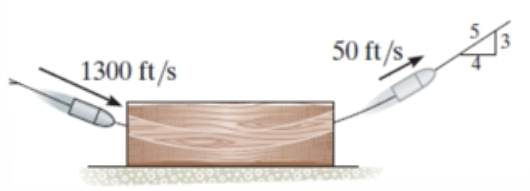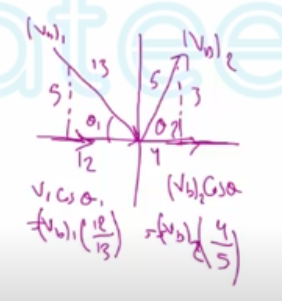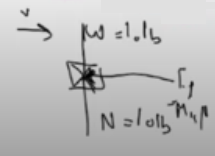Need Help?

Subscribe to Dynamic

###### \${selected_topic_name}
• Notes
• Comments & Questions

$\begin{array}{l}{\text { 15- 41. A } 0.03-\text { lb bullet traveling at } 1300 \mathrm{ft} / \mathrm{s} \text { strikes the }} \\ {10 \text { -lb wooden block and exits the other side at } 50 \mathrm{ft} / \mathrm{s} \text { as }} \\ {\text { shown. Determine the speed of the block just after the }} \\ {\text { bullet exits the block, and also determine how far the block }} \\ {\text { slides before it stops. The coefficient of kinetic friction }} \\ {\text { between the block and the surface is } \mu_{k}=0.5 \text { . }}\end{array}$$W_{b}=0.03l b$

$\left(V_{b}\right)_{1}=1300 ft / s$

$\left(V_{b}\right)_{2}=50ft/s$

$W_{b k}=10l{b}$

$\mu_{k}=0.5$

$\Sigma{m_{1}} v_{1}=\Sigma m_2 v_2 (\stackrel{+}{\rightarrow})$$\left(\frac{w_{b}}{g}\right)\left(v_{b}\right)_{1}\left(\frac{12}{13}\right)+\left(\frac{w_{b k}}{g}\right) V_{b k}$

$=\left(\frac{w_{bk} }{g}\right) v_{2} b_{k}+\left(\frac{w_{b}}{2}\right) v_{2} b\left(\frac{4}{5}\right)$

$∴\left(\frac{0.03}{32.2}\right)(1300)\left(\frac{12}{13}\right)+0=\left(\frac{10}{32.2}\right) V_{2} b_{k}+\left(\frac{ 0 .03}{32.2}\right)50 (\frac{4}{5})$

$V_{2 b k}=3.48 \mathrm{ft} / \mathrm{s}$

$T_{1}+\Sigma U_{1 \rightarrow 2}=T_{2}$$\frac{1}{2} m v_{1}^{2}-F_{f} d=\frac{1}{2} m v_{2}$

$\frac{1}{2}\left(\frac{10}{32.2}\right)(3.48)^{2}-(0.5)(10)(d)=0$

$d=0.376 \mathrm{ft}$

$\begin{array}{l}{\text { 15-58. Disk } A \text { has a mass of } 250 \mathrm{g} \text { and is sliding on a }} \\ {\text { smooth horizontal surface with an initial velocity }} \\ {\left(v_{A}\right)_{1}=2 \mathrm{m} / \mathrm{s} . \text { It makes a direct collision with disk } B, \text { which }} \\ {\text { has a mass of } 175 \mathrm{g} \text { and is originally at rest. If both disks are }} \\ {\text { of the same size and the collision is perfectly elastic }(e=1) \text { , }} \\ {\text { determine the velocity of each disk just after collision. Show }} \\ {\text { that the kinetic energy of the disks before and after collision }} \\ {\text { is the same. }}\end{array}$

$m_{A}=250g$

$\left(v_{A}\right)_{1}=2 \mathrm{m} / \mathrm{s}$

$m_{B}=175g$

$\left(v_{B}\right)_{1}=0$

$e=1$

$+m_{A}\left(v_{A}\right)_{1}+m_ B\left(v_{B}\right)_{1}=m_{A}\left(v_{A}\right)_{2}+m_{B}\left(v_{B}\right)_{2}$

$\left(\frac{250}{1000}\right) 2+\left(\frac{175}{1000}\right) * 0=\left(\frac{250}{1000}\right)(v_A)_{2}+\left(\frac{175}{1000}\right)(v_B)_{2}$

$∴ 0.5=(0.25)\left(v_{A}\right)_{2}+(0.175)\left(v_{B}\right)_{2} \rightarrow (1)$

$e=\frac{(v_B)_{2} -\left(v_{A}\right)_{2}}{\left(v_{A}\right)_{1}-\left(v_{B}\right)_{1}}=1=\frac{\left(\left.v_{B}\right)_{2}-(v_{A}\right)_{2}}{2-0} \Rightarrow 2=-\left(v_{A}\right)_{2}+\left(v_{B}\right)_{2} \rightarrow (2)$

$\left(v_{A}\right)_{2}=0.353 \mathrm{m} / \mathrm{s}$ , $\left(v_{B}\right)_{2}=2.35 m/s$

$T_{1}=\frac{1}{2} m\left(v_{A}\right)_1^{2}+\frac{1}{2} m(v_ B)_{1}^{2}=\frac{1}{2}(0.25) 2^{2}+\frac{1}{2}(0.175) 0=0.5 J$

$T_{2}=\frac{1}{2}(0.25)(0.353)^{2}+\frac{1}{2}(0.175)(2.35)^{2}=0.5J$

$∴ T_{1}=T_2$

No comments yet